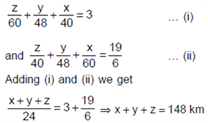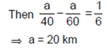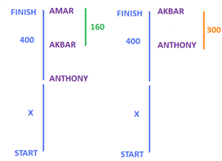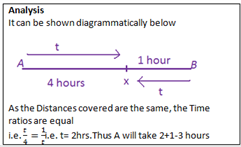# Time-Speed-Distance: Solution

The solutions to time speed distance: questions are given here. The solutions are detailed with proper explanations of each concept. Also, the best approach is given here that would help to solve the related questions much faster and efficiently.

### TIME SPEED DISTANCE – SOLUTIONS

1) Option (d)

As the Distance covered is same with both the Speeds, the average Speed will be the harmonic mean of the individual Speed.

200/9m/s = (200*3600)/((9*1000) ) = 80 km/h

So, average Speed = (2*a*b)/(a + b) = (2 * 80 * 120)/(80 + 120) = 96 km/h.

2) Option (c)

Distance = Speed × Time

As Distance covered in both the cases is constant, Speed will be inversely proportional to time.

So, if Time by bus: Time by train = 2: 1, Speed of bus: Speed of train = 1: 2

Speed of bus is 40 km/hr, so Speed of train is 80 km/hr.

3) Option (a)

Distance = Speed x Time

In both the cases, Speed is constant so Distance will be proportional to the time.

Vivek takes 20% more than Bharath. So, assume that Bharath takes 100 minutes then Vivek will take 20% more i.e. 20 minutes more, so 120 minutes. So, the ratio of Time taken by Vivek and Bharath = 120/100= 6/5. Distance is proportional to the Time taken, so ratio of Distance of Vivek’s House and Bharath’s House = 6/5, Bharath’s house is at a Distance of 30 km, so Vivek’s house will be at the Distance of (6/5)*30 = 36 km.

4) Option (d)

#### Method 1:

Suppose the normal Time taken is ‘t’ then when he is late by 1 hour, Time taken by him = ‘t – 1’.

Normal Speed = 60/t ,Speed when he wants to reach in t – 1 Time = 60/(t – 1)(60/(t-1))– (60/t) = 5? t2 – t – 12 = 0 ? (t – 4) (t + 3) = 0 ? t = 4 and t = – 3 (-3 is not possible)So, normal Time taken = 4 hours. Hence option (d).

#### Method 2: Reverse Gear Approach

Take a middle answer option, say 5 hours Normal Speed = 60/5 = 12 kmph Speed increased to 12+5=17 kmph. 60/17 = 3.53 hours,

the difference is more than 1 hour. This is not the answer. We can also eliminate 6 hours, as that will result in a higher Time difference.

Answer is either 3 or 4 hours(answer is close to 5) Trying for 4 hours, Normal Speed= 60/4 = 15 km/h

Speed increased to 20 km/h 60/20=3. 4-3 =1. This is the answer.

5) Option (c)

Sajesh increases his Speed from 12 km/hr to 15 km/hr, so increase in Speed = 3 km/hour 1/4. In both the cases, Distance covered remains the same. So, applying constant product rule: His Speed increases by 1/4 so Time will decrease by 1/5.

Here Time is decreasing by 1 hour and 1 hour is 1/5 of the actual Time taken. So, actual Time taken = 5 hours. So, Distance between Office and House = 5 * 12 = 60 kms. Hence option (c). Alternatively, you can also check the answer options.

(a) Distance = 40 kms, actual Speed = 12. Actual Time taken = 40/12. New Time taken = 40/15.

(40/12)– (40/15)≠ 1

(b) Distance = 50 km, actual Speed = 12. Actual Time taken = 50/12. New Time taken = 50/15. (50/12)– (50/15)≠1

(c) Distance = 60 kms, actual Speed = 12. Actual Time taken = 60/12= 5 hrs. New Time taken = 60/15 = 4 hrs.

Difference = 1 hour.

6) Option (b)

Let x,y and z be the Distances uphill, on flat ground and downhill respectively. Then7) Option (a)

Let AC be 3 km more than DE. ThenThus, if the Distance is decreased by 20 km, the Time taken will be the same.

8) Option (d)

Their Speeds are not given. So the ratio of the Time taken cannot be determined.

9) Option (d)

There is no traveling Distance because they can travel anywhere in the path. So the ratio of their Speeds cannot be determined i.e., for example, if player P2 will take the path N-J-F-B, or N-d-a-B etc. can never be determined.

10)Option (c)

If you observe the options carefully, you can decide which answer option to start with. Since the fraction 5/6 is involved. Start with an option which is a multiple of 6; option (b) and option (c) Now one has 2 Distances 20 km and 22 km A’s original Speed = 6kmph. In 2 hours he will travel 12 km B’s original Speed= 4 kmph. In 2 hours he will travel 8 km 12+8=20 and not 22. Here itself, you can mark the answer as option (c) Verifying A’s new Speed= 5 kmph B’s new Speed = 5 kmph After 1 hour A travels 5 km, B travels 0 After 2 hours A travels 10 km (total), B travels 5. Total =15 km. They thus meet after 2.5 hours.

11) Option (a)

It is given that the platoon and the last person moved with uniform Speed. Also, they both moved for the identical amount of Time. Hence, the ratio of the Distance they covered – while person moving forward and backward – are equal. Let’s assume that when the last person reached the first person, the platoon moved X meters forward. Thus, while moving forward the last person moved (50+X) meters whereas the platoon moved X meters. Similarly, while moving back the last person moved [50-(50-X)] = X meters whereas the platoon moved (50-X) meters. Now, as the ratios are equal

(50+X)/X = X/(50-X) (50+X)*(50-X) = X*X Solving, X=35.355 meters Thus, total Distance covered by the last person = (50+X) + X = 2*X + 50 = 2*(35.355) + 50 = 120.71 meters =120 m (approximately).

12) Option (b)

Using the concept of ratios of Speed (Speed directly proportional to Distance), we can easily arrive at the totalDistance. The images below give a picture of the Distances when Amar and Akbar finish the raceLength of the race track = 400 +x The ratio of Akbar and Anthony’s Speed can be arrived at as (240+x)/x = (400+x)/(100+x)

Now go from answer options, answer will be option (b) 800 m Verifying, x = 400 640/400 = 800/500. Thus, answer is option (b).

13) Option (c)

The ratio of Speeds of A and B= 3:1, This means that when A makes 1.5 rounds, B makes 0.5 round. For B to complete the race of 15 rounds, it would take him 15/0.5 x 5 =150 minutes.

14) Option (c)

Solve the question using assumption If B’s Speed is 3, then A’s Speed is 3+(3/3) = 4 If A travels 12 km, B travels 6 km Ratio of Time taken = A:B = 3:2. Option (c).

15) Option (b)

In 2/3 of an hour A travels 10 km in 40 min B travels 8 km in 40 min After one hour, A would have still traveled only 10 km and B would have traveled 20 km, their relative Speed = 15-12= 3 km/hr A gains this 10 km in 10/3 =3.33 hours.

16) Option (b)

the total Distance traveled before they come to a halt can be calculated as 40(1+1/2+1/4+1/8…)/6 = 40((1/(1-1/2))/6)=13.33 km They cover a Distance of 750 m for the first time they meet, and subsequently cover a Distance of 1500 m each Time they meet, hence if they meet 8 Times, then the Distance covered will be 1500 * 8 + 750 = 12750, which is lesser than 13,333.33. If they meet 10 Times then the Distance would be 14250>13333.33. Hence they meet 9 Times.

17) Option (d)

Let T be Time Katrina takes to make 25 steps. Then Katrina takes 3T to make 75, and Priyanka takes 2T to make 150. Suppose the escalator has N steps visible and moves n steps in Time T. Then Priyanka covers N + 2n = 150, N – 3n = 75. Hence N = 120, n = 15 => Choice (d) is the right answer.

18) Option (c)

Let the escalator moves x steps when A walks down 90 steps. Total number of steps on a stationary escalator = x + 90. When A takes 90 steps, B should have taken 60 steps and the escalator x steps. So when B takes 80 steps, the escalator should have taken 4/3*x steps. So, 4/3*x + 80 = x + 90 = Total number of steps in the escalator when it is stationary. So x = 30. Hence, total number of steps = 120. By the Time they meet, together they will 120 steps in the ratio 3:2. i.e 72 and 48 steps. So, the difference is 24. Option (d).

19) Option (b)

Time taken by Ship 1 = 3x/40 hr Time taken by Ship 2 = 2x/60 hr 3x/40–2x/60= 1

10x=240 x=24 Distances traveled are 48 & 72= 120.

20) Option (d)

Consider that Ishan’s journey will end when the group reaches the end of the walkway (as long as he catches up with the crowd before the walkway ends). When he steps on the walkway, the crowd is 180 metres from the end. The walkway travels this Distance in (180/3) = 60 seconds, and Ishan’s average rate of movement is 300/60 = 5 metres per second.

21) Option (d)

Let x be the number of hours Sana walks at 10kmph. x will be the no. of hours Peter and Gavin traveled by car at 50kmph. Let x1 be the number of hours that Gavin walks till Peter reaches Sana. Let x2 be the number of hours when Peter and Sana travel in the car till the destination. The total Distance traversed by each of them can be evaluated as follows

Peter = 50x-50*1+50*2=200——————(1)

Sana= 10x+10*1+50*2=200—————— (2)

Gavin = 50x+10*1+10*2=200—————–(3)

Solving we get t1= 2 hours, t=3 hours, t2=3 hours. Total = 8 hours.

22) Option (c)

This problem can be solved by noticing that the Speeds are in a ratio of 2:1. The Distances covered will, therefore, be in a ratio of 2:1. When they meet for the 8th Time, they would have covered the entire Distance of the track 8 Times = 8 (2pr) .Anu would have covered 8 Times the Distance and Varsha 4 Times the Distance (Total= 12, ratio 2:1) Difference = 4(2pr) = 4x 2 x (22/7)x(140/2)= 1760.

23) Option (d)

1400 m track is divided into 7 parts of 200 m each. The Speeds of the two bikes are in a ratio of 3:4, each time they meet, they will cover a Distance in the ratio of 3:4 They will thus meet for the first Time at 3/7th the Distance which will happen at point 4 is 600 m. The second time they will meet at the starting point. 3rd Time at point 4… and so on.so the 15th Time, they meet at 600 m which is 800 m from A.

24) Option (c)

Cost incurred (maximum ) a Speed x capacity x cost/km Required ratio= (7x* 5x* 3x)/( 4x* 2x* 2x)=105/16.

25) option (a)

As the Distance covered is constant in both the cases, the Time taken will be inversely proportional to the Speed. In the problem, Speed of Amit and Aman is in ratio 60: 90 or 2:3. So the ratio of the Time taken by Amit to that taken by Aman will be in the ratio 3:2. So if Amit takes 9 hrs, Aman will take 6 hrs.

26) Option (b)

This is an instance of Case 1 as illustrated above. When Ram meets Shyam for the third Time, they together would have covered a Distance of 5d, i.e 5x30m = 150 m. Ratio of Speed of Ram and Shyam = 2:1, so the total Distance traveled by them will also be in the ratio 2:1 as the Time taken is constant. So the Distance traveled by Ram will be 2/3 x150= 100 m.

27) Option (d)

A takes 1 hour after meeting and B takes 4 hours after meeting. Hence Time traveled before meeting = v1.4 = 2 hours Time taken by A= 2+1 = 3 hoursStudy tuned with Byju’s for a better understanding of topics and proper preparation for the CAT exam, At Byju’s candidates, are also provided with the CAT Syllabus and other free Study materials to learn more effectively.